## Tubing Length Change due to Buckling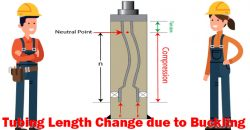When tubing is freely suspended, it can be buckled by an upward force applied at the bottom of tubing. A section of tubing exposed to compression force will have a chance of being buckled. However, a part which is under tension will not face a buckle issue.

The neutral point is the boundary below which buckling can possibly be occurred and above which buckling will not happen.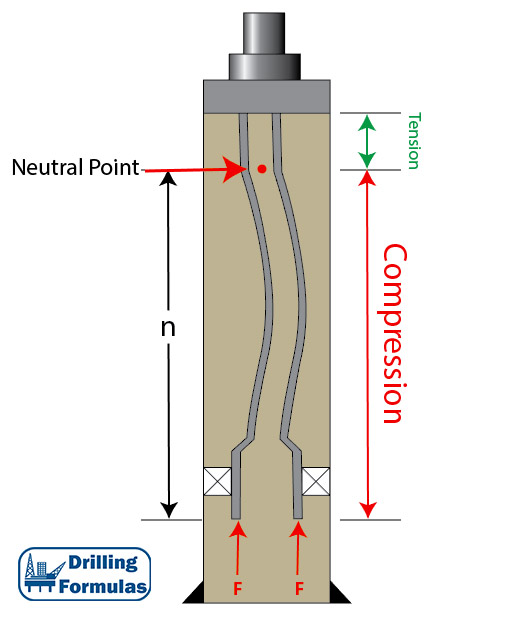Figure 1 – Wellbore Diagram with Tubing Buckling Due to Compression Force

## Tubing Length Change due to Thermal LoadDifference in temperature causes steel to contract or expand. If tubing is free to move, length of the tubing will be either longer or shorter due to thermal expansion. On the other hand, if the tubing is not free to move, there will be a change in axial force due to the temperature effect.

Figure 1 illustrates an increase in length due to heat and Figure 2 demonstrates a decrease in length because of cooling.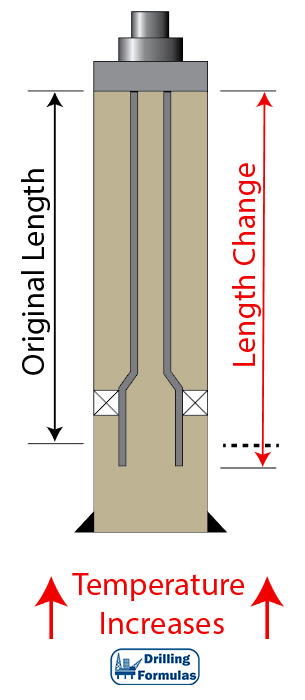Figure 1 – Tubing Lengthen by Temperature Increase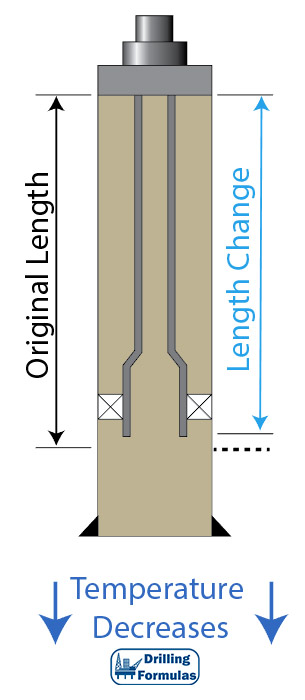Figure 2 – Tubing Shorten by Temperature Decrease

## Tubing Length Change due to Ballooning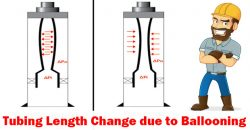Ballooning is a change in average pressure which causes a radial contraction or swelling. If the tubing is free to move, the length of tubing will be either longer or shorter. If tubing cannot be moved, stress will be created in the tubing body.

Figure 1 illustrates ballooning of tubing. This will happen when internal pressure is higher than external pressure. Tubing will shorten if the tubing is free to move. If the tubing cannot be moved, ballooning will create a tension force on the packer.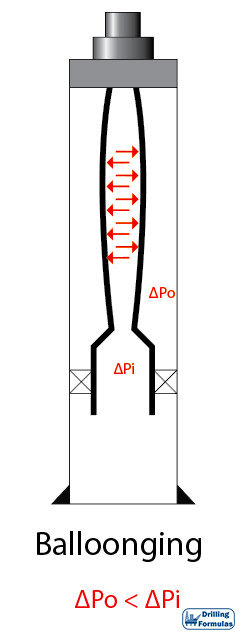Figure 1 – Ballooning

## Tubing Length Change due to Piston Effect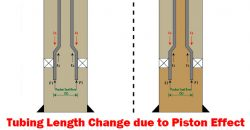This article demonstrates a piston effect and how it will change the length of tubing with full detailed calculations.

For the analysis, Lubinski’s sign conventions are used.

Compression force = ⇑(+)

Tensile force = ⇓ (-)

Shorten in Length = (-)

Elongate in length = (+)

Note: The tubing is free to move.

Please see this in the reference section to get a full paper.

Total force change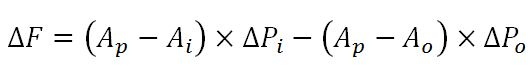Total length change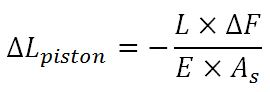Continue reading